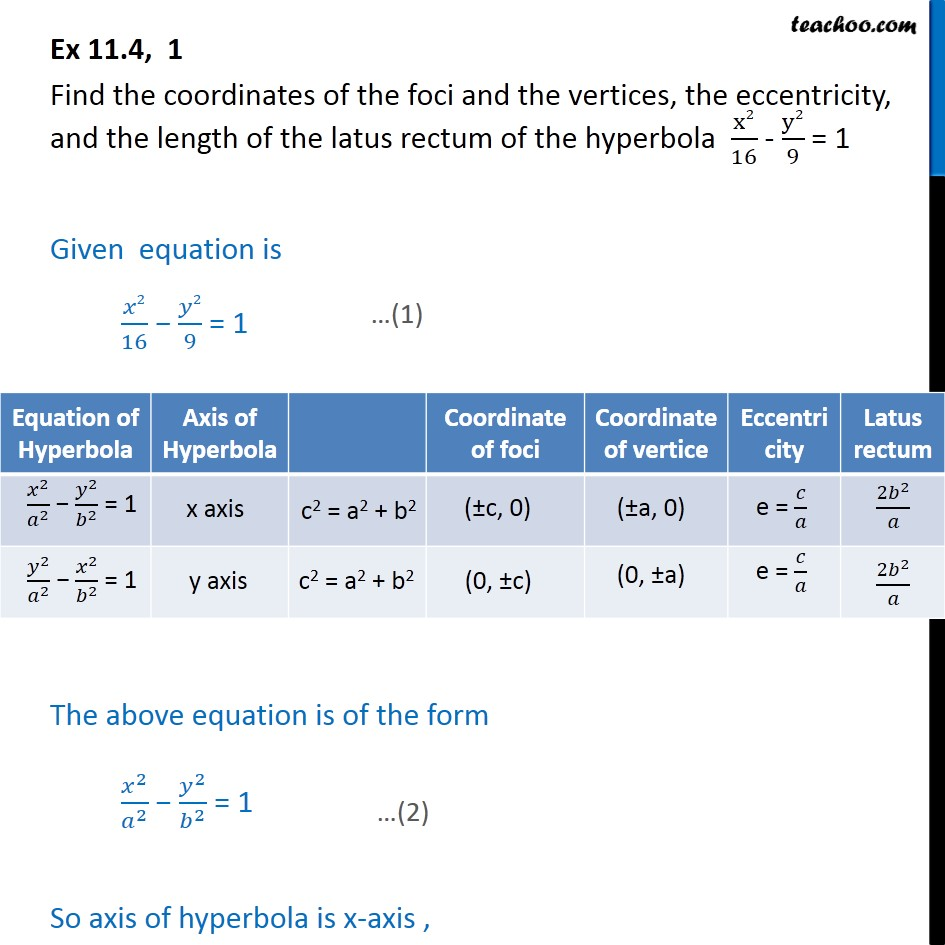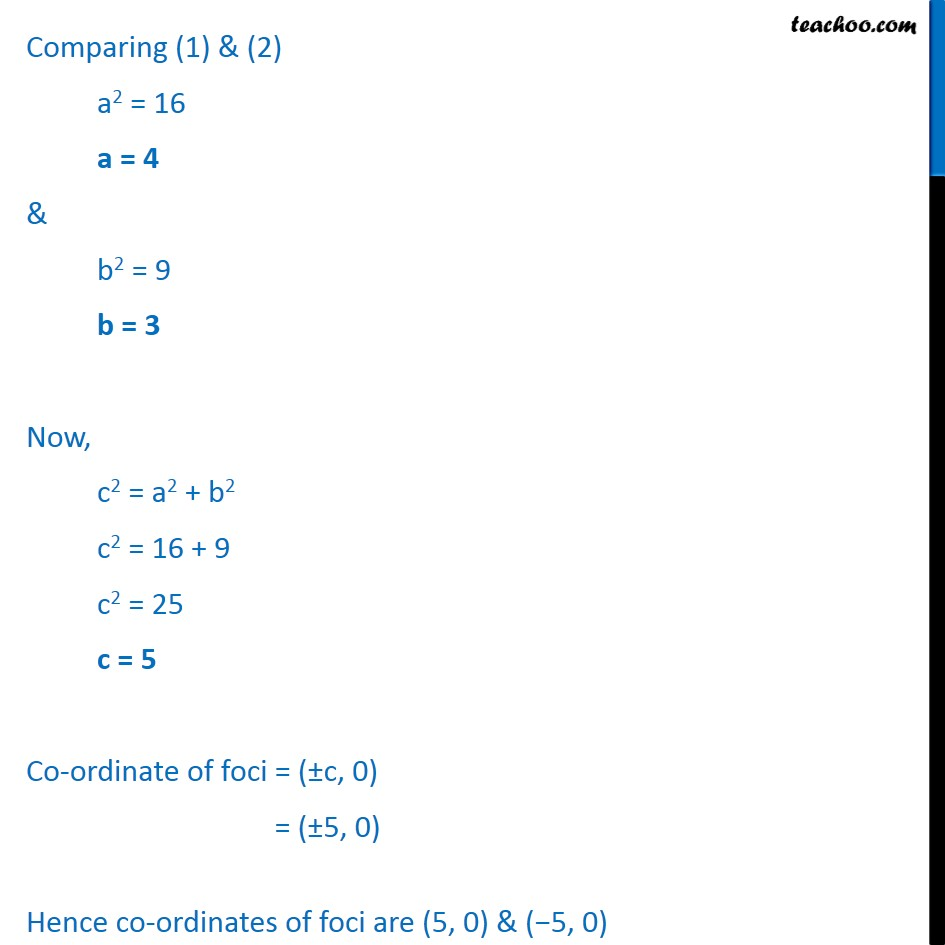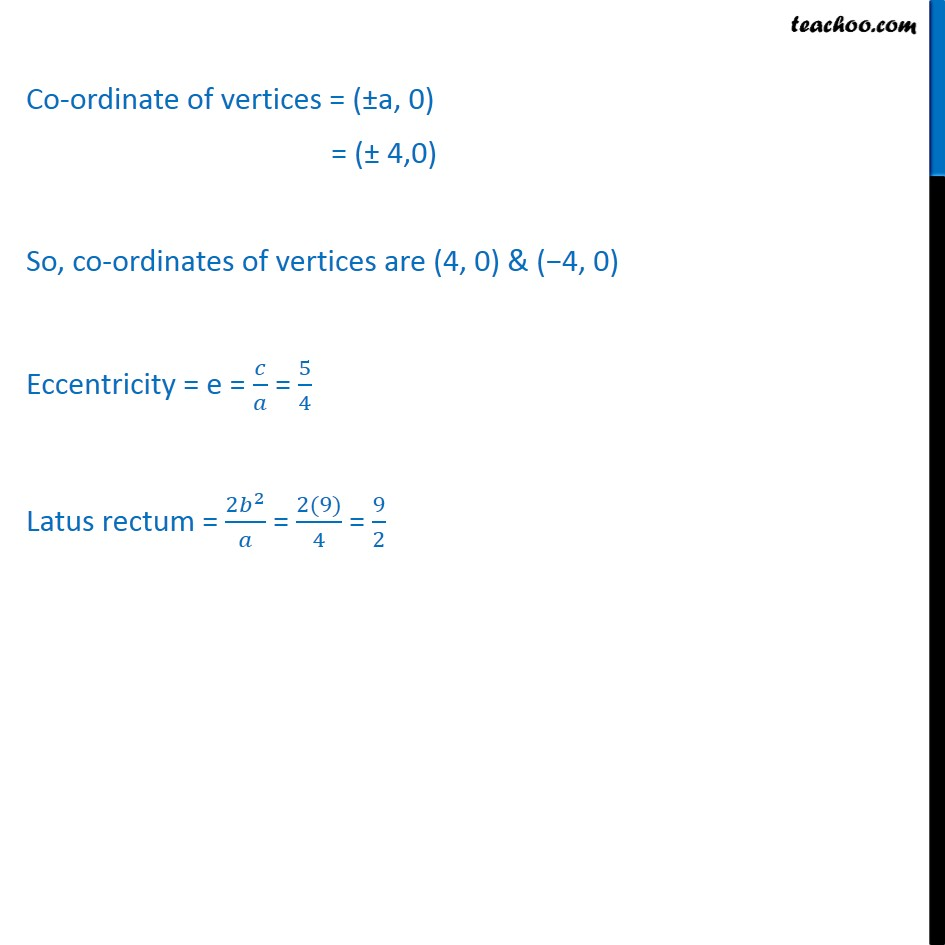Ex 10.4

Chapter 10 Class 11 Conic Sections
Serial order wiseLearn in your speed, with individual attention - Teachoo Maths 1-on-1 Class

### Transcript

Ex 10.4, 1 Find the coordinates of the foci and the vertices, the eccentricity, and the length of the latus rectum of the hyperbola x2 16 - y2 9 = 1 Given equation is 2 16 2 9 = 1 The above equation is of the form 2 2 2 2 = 1 So axis of hyperbola is x-axis , Comparing (1) & (2) a2 = 16 a = 4 & b2 = 9 b = 3 Now, c2 = a2 + b2 c2 = 16 + 9 c2 = 25 c = 5 Co-ordinate of foci = ( c, 0) = ( 5, 0) Hence co-ordinates of foci are (5, 0) & ( 5, 0) Co-ordinate of vertices = ( a, 0) = ( 4,0) So, co-ordinates of vertices are (4, 0) & ( 4, 0) Eccentricity = e = = 5 4 Latus rectum = 2 2 = 2(9) 4 = 9 2